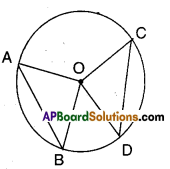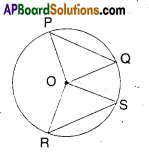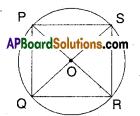# AP Board 9th Class Maths Solutions Chapter 12 Circles Ex 12.2

AP State Syllabus AP Board 9th Class Maths Solutions Chapter 12 Circles Ex 12.2 Textbook Questions and Answers.

## AP State Syllabus 9th Class Maths Solutions 12th Lesson Circles Exercise 12.2Question 1.
In the figure, if AB = CD and ∠AOB = 90° find ∠COD.Solution:
‘O’ is the centre of the circle.
AB = CD (equal chords from the figure)
∴ ∠AOB = ∠COD
[ ∵ equal chords make equal angles at the centre]
∴ ∠COD = 90° [ ∵ ∠AOB = 90° given]Question 2.
In the figure, PQ = RS and ∠ORS = 48°.
Find ∠OPQ and ∠ROS.Solution:
‘O’ is the centre of the circle.
PQ = RS [given, equal chords]
∴∠POQ = ∠ROS [ ∵ equal chords make equal angles at the centre]
∴ In ΔROS
∠ORS + ∠OSR + ∠ROS = 180°
[angle sum property]
∴ 48° + 48° + ∠ROS = 180°
[ ∵ OR = OS(radii); ΔORS is isosceles]
∴ ∠ROS = 180° – 96° = 84°
Also ∠POQ = ∠ROS = 84°
∴ ∠OPQ = ∠OQP
= $$\frac { 1 }{ 2 }$$[180°-84°] = 48°Question 3.
In the figure, PR and QS are two diameters. Is PQ = RS ?Solution:
‘O’ is the centre of the circle.
[ ∵ PR, QS are diameters]
OP = OR (∵ radii) .